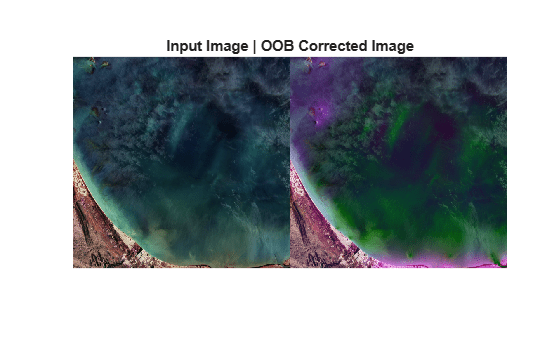# correctOOB

Correct out-of-band effect using sensor spectral response

## Syntax

``newhcube = correctOOB(hcube,spectralResponse)``
``[newhcube,oobEffect] = correctOOB(hcube,spectralResponse)``
``[___] = correctOOB(hcube,spectralResponse,'RegionMask',mask)``
``newhcube = correctOOB(___,'BlockSize',blocksize)``

## Description

example

````newhcube = correctOOB(hcube,spectralResponse)` corrects the out-of-band (OOB) effect in the input satellite data by using the sensor's spectral response characteristics. This method is suitable for OOB correction in multispectral satellite data.Use this function to correct OOB effects over different regions such as clear waters, turbid waters, green vegetation, sand, and soil. This method gives best results if the input data is compensated for Rayleigh and aerosol scattering. To measure the OOB effect on scenes with large water bodies, you must first compute the water leaving radiance spectra from the input satellite data.```

example

````[newhcube,oobEffect] = correctOOB(hcube,spectralResponse)` also returns the relative OOB effect for each spectral band.```

example

````[___] = correctOOB(hcube,spectralResponse,'RegionMask',mask)` specifies region mask by using the name-value pair argument `'RegionMask'`. The region mask indicates the homogeneous regions in the input satellite data.```
````newhcube = correctOOB(___,'BlockSize',blocksize)` specifies the block size for block processing of the hyperspectral data cube by using the name-value pair argument `'BlockSize'`. You can specify the `'BlockSize'` name-value pair argument in addition to the input arguments in the previous syntaxes. The function divides the input image into distinct blocks, processes each block, and then concatenates the processed output of each block to form the output matrix. Hyperspectral images are multi-dimensional data sets that can be too large to fit in system memory in their entirety. This can cause the system to run out of memory while running the `correctOOB` function. If you encounter such an issue, perform block processing by using this syntax.For example, `correctOOB(hcube,spectralResponse,'BlockSize',[50 50])` divides the input image into non-overlapping blocks of size 50-by-50 and then performs out-of-band correction on each block. NoteTo perform block processing by specifying the `'BlockSize'` name-value pair argument, you must have MATLAB® R2021a or a later release. NoteThis function requires the Image Processing Toolbox™ Hyperspectral Imaging Library. You can install the Image Processing Toolbox Hyperspectral Imaging Library from Add-On Explorer. For more information about installing add-ons, see Get and Manage Add-Ons. ```

## Examples

collapse all

Read multispectral data into the workspace.

`hcube = hypercube('LC08_L1TP_097070_20201101_20201101_01_cropped.dat');`

Convert the pixel values from digital numbers to top of atmosphere (TOA) radiance values.

`hcube = dn2radiance(hcube);`

Estimate remotely sensed water reflectance.

`[rrshcube,mask] = rrs(hcube);`

Read the sensor spectral response.

```spectralResponse = readtable('spectralResponse.txt'); spectralResponse = table2array(spectralResponse);```

Perform OOB correction using the clear-water pixels specified by the region mask.

`[newhcube,oobEffect] = correctOOB(rrshcube,spectralResponse,'RegionMask',mask);`

Estimate RGB images of the input and the OOB corrected output data. Increase the image contrast by applying contrast stretching.

```imgIn = colorize(hcube,'Method','rgb','ContrastStretching',true); imgOut = colorize(newhcube,'Method','rgb','ContrastStretching',true);```

Display the input and the OOB corrected output images.

```figure montage({imgIn,imgOut}) title('Input Image | OOB Corrected Image')```## Input Arguments

collapse all

Input satellite data, specified as a `hypercube` object. The functions reads the data cube from the `DataCube` property of the object. The data cube is of size M-by-N-by-C. C is the number of spectral bands in the input satellite data.

Sensor spectral response, specified as a matrix or a table. The size of the matrix or the table must be K-by-C+1. The first column of the matrix or table contains the wavelength values and the spectral resolution is 1 nm.

You can download the spectral response for different sensors from https://oceancolor.gsfc.nasa.gov/docs/rsr/rsr_tables/.

Region mask indicating homogeneous regions, specified as a matrix of size M-by-N. The region mask is a binary image with intensity values 0 and 1. The regions with intensity value 1 corresponds to the homogeneous regions in the input satellite data.

Example: `correctOOB(hcube,spectralResponse,'RegionMask',mask)`

Data Types: `logical`

Size of the data blocks, specified as a 2-element vector of positive integers. The elements of the vector correspond to the number of rows and columns in each block, respectively. The size of the data blocks must be less than the size of the input image. Dividing the hyperspectral images into smaller blocks enables you process large data sets without running out of memory.

• If the `blocksize` value is too small, the memory usage of the function reduces at the cost of increased execution time.

• If the `blocksize` value is large or equal to the input image size, the execution time reduces at the cost of increased memory usage.

Example: `'BlockSize',[20 20]` specifies the size of each data block as 20-by-20.

## Output Arguments

collapse all

Out-of-band corrected data, returned as a `hypercube`.

Out-of-band effect for each band, returned as a C-element vector. The out-of-band effect for each spectral band is measured as the relative difference between the values of homogeneous region pixels in the input data `hcube` and the corrected data `newhcube`.

## Version History

Introduced in R2020b